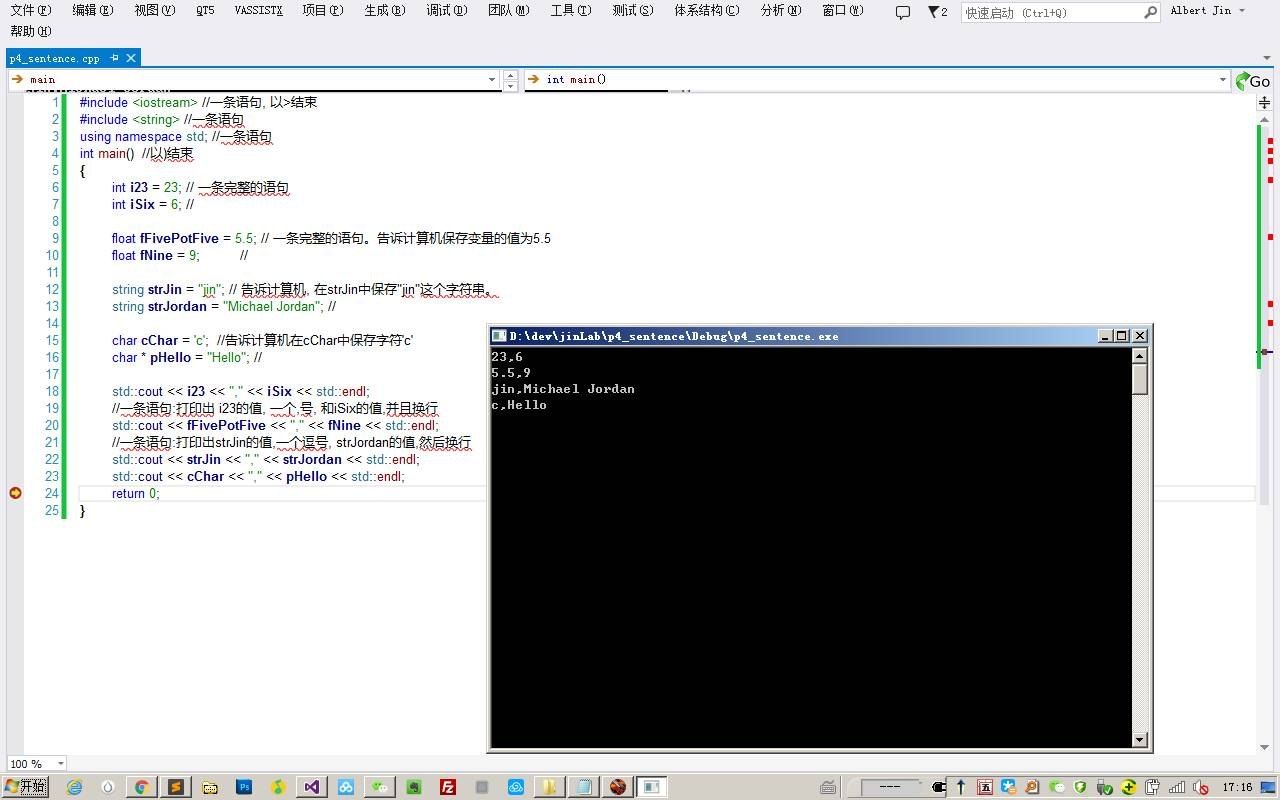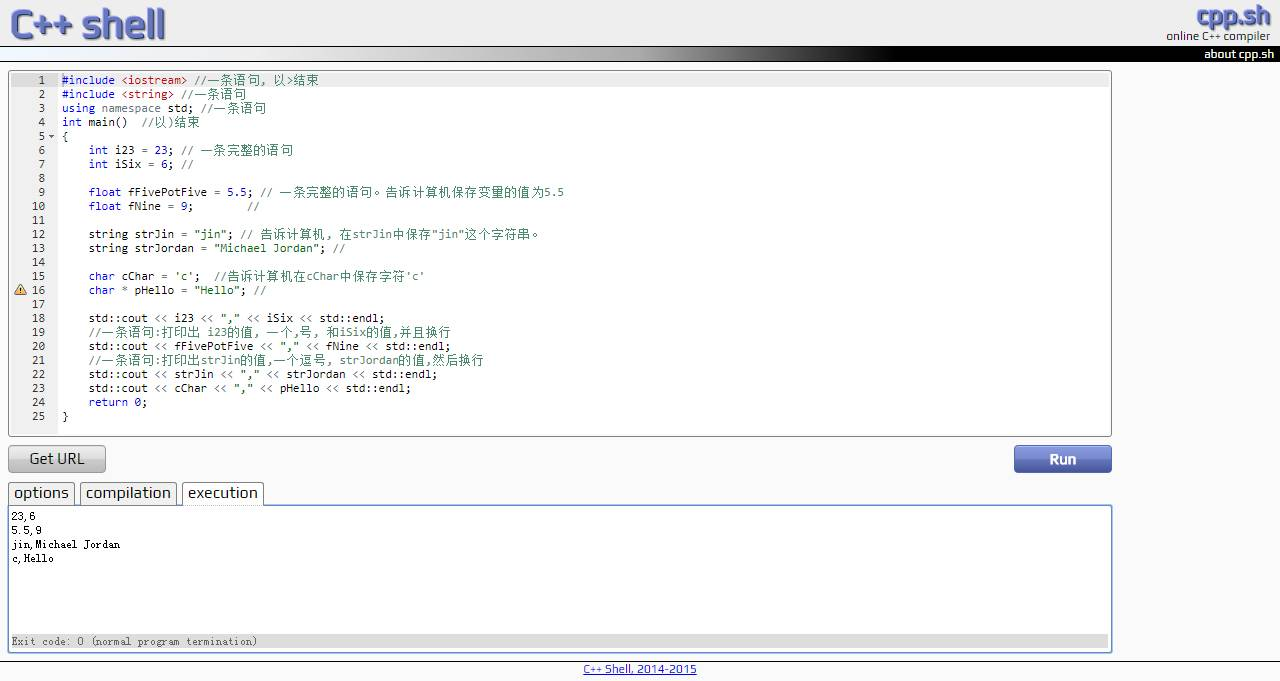# Tag: 小宁聊编程系列

## 小宁和你聊编程之四: 语句篇

Posted by – September 26, 2017

``````int iFive = 5; //int(整型)变量iFive的值为5.
``````
``````string strJ="jin"; //字符串strJ的值为"jin"
``````

1.什么是语句?

.表达完整意思: 就像你说话得说完整，要不然大家会听不明白。

.一句话: 这句话通常以;(绝大多数情况) 或者右大括号}, 或者>, 或者以) 结束.

2.语句的应用?(举个例子)

``````int i23=23; //语句,以int开始, 以;结束.
``````
``````int iSix=6; //一条语句,以int始, 以;终.
``````

``````string strJ="j"; //语句:以string始,以;终
``````
``````string strJor="Mi"; //语句:以string开始,以;终
``````

``````char cCh='c'; //语句:以char始,以;终.
``````
``````char * pHi="Hi"; //语句:以chra始,以;终.
``````

``````; //以;结束的语句。什么也不做.
``````

3.语句的实践?

``````#include <iostream> //一条语句, 以>结束
#include <string> //一条语句
using namespace std; //一条语句
int main()  //以)结束
{
int i23 = 23; // 一条完整的语句
int iSix = 6; //

float fFivePotFive = 5.5; // 一条完整的语句。告诉计算机保存变量的值为5.5
float fNine = 9;        //

string strJin = "jin"; // 告诉计算机, 在strJin中保存"jin"这个字符串。
string strJordan = "Michael Jordan"; //

char cChar = 'c';  //告诉计算机在cChar中保存字符'c'
char * pHello = "Hello"; //

std::cout << i23 << "," << iSix << std::endl;
//一条语句:打印出 i23的值, 一个,号, 和iSix的值,并且换行
std::cout << fFivePotFive << "," << fNine << std::endl;
//一条语句:打印出strJin的值,一个逗号, strJordan的值,然后换行
std::cout << strJin << "," << strJordan << std::endl;
std::cout << cChar << "," << pHello << std::endl;
return 0;
}
``````https://press.one/p/v?s=4f904e6be71ca0f004bbd3a4fd1494a519c0260c52de9c442543275a95c6252b305ce2617b228fcaaac056f3c7ece7641b582c8d28317d7629cfbf29868178780&h=f4eb7f73b61362dd7677e85ef7e4871c88fb6006c592ae623af40637a1660730&a=e49b24f3c39653372223aeac8798cf3c47ca56f5&f=P1&v=3